Publication date: 08/13/2020

## Summary Statistics

This section contains statistical details for specific statistics in the Summary Statistics report.

### Mean

The mean is the sum of the nonmissing values divided by the number of nonmissing values. If you assigned a Weight or Freq variable, the mean is computed by JMP as follows:

1. Each column value is multiplied by its corresponding weight or frequency.

2. These values are added and divided by the sum of the weights or frequencies.

### Std Dev

The standard deviation measures the spread of a distribution around the mean. It is often denoted as s and is the square root of the sample variance, denoted s2.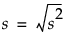where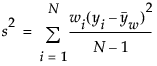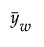= weighted mean

### Std Err Mean

The standard error mean is computed by dividing the sample standard deviation, s, by the square root of N. In the launch window, if you specified a column for Weight or Freq, then the denominator is the square root of the sum of the weights or frequencies.

### Skewness

Skewness is based on the third moment about the mean and is computed as follows: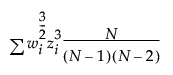where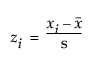and wi is a weight term (= 1 for equally weighted items).

### Kurtosis

Kurtosis is based on the fourth moment about the mean and is computed as follows: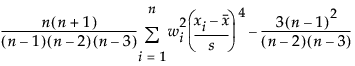where wi is a weight term (= 1 for equally weighted items). Using this formula, the Normal distribution has a kurtosis of 0. This formula is often referred to as the excess kurtosis.